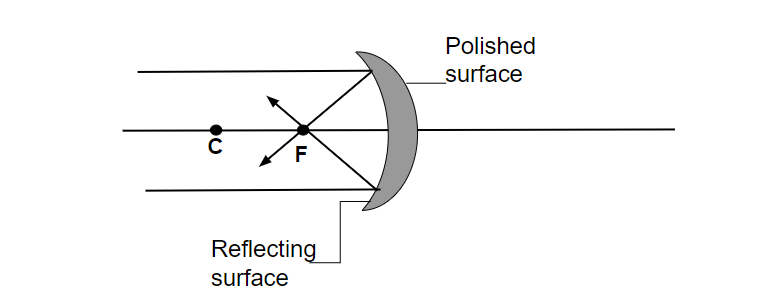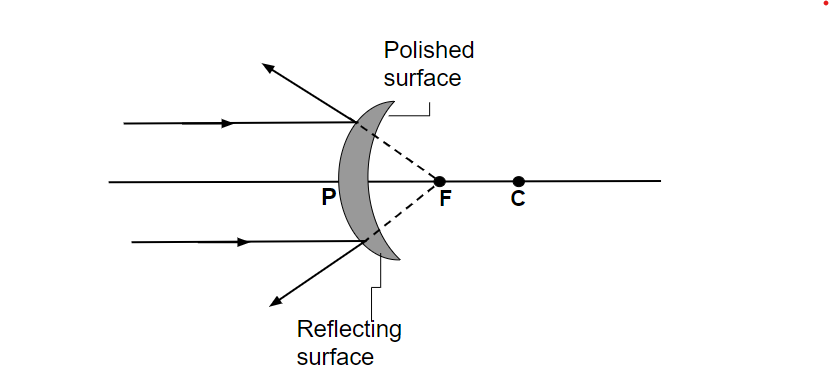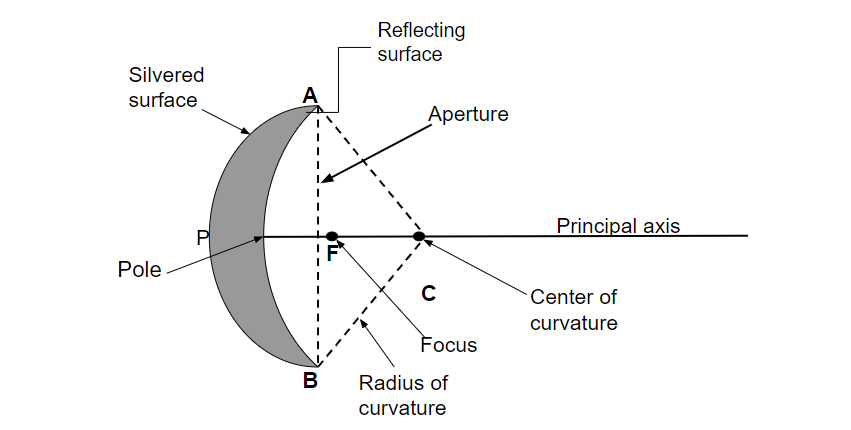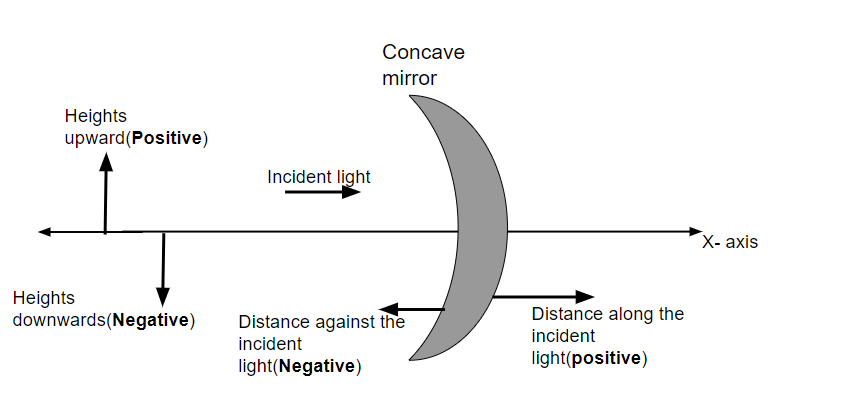GeeksforGeeks App
Open AppBrowser
Continue

## Related Articles

You might have observed that the image formed in the side view mirror of a car is smaller while the image formed in the rear-view mirror is larger than the actual size of the objects. The dentist uses a small mirror to examine the teeth of a patient. You might have also observed that for shaving purposes different mirrors are used. So, the different spherical mirrors are used for different purposes. We will discuss the spherical mirror in this chapter.

Spherical mirror is a type of mirror whose reflecting surfaces is the parts of a hollow sphere of glass.

### Types of Spherical Mirror

On the basis of the direction of reflecting surface and reflection, they are of two types namely-

1. Concave mirror, and
2. Convex Mirror.

Concave Mirror

The type of spherical mirror in which reflection of light takes place from the side which is curved inward and the outer bulged surface is silvered polished is called a concave mirror. It is also called the converging mirror. It converges the parallel beam of light at focus(F). The shaving mirror and the mirror used by a dentist are examples of concave mirrors.Concave mirror.

Properties of Concave Mirror

• A ray of light parallel to the principal axis will pass through the focus after reflection.
• A ray passing through the principal focus(F) becomes parallel to principal axis.
• A ray passing through the center of curvature will take the same path after reflection.
• For any ray incident at any angle at the pole(P), the reflected ray follows the law of reflection (angle of incidence = angle of reflection).
• The  nature of image formed by concave mirror can be real, virtual, erect, inverted.
• The size of the image can be smaller, larger or equal to the object.

Applications of Concave Mirror

• Shaving mirror
• Torch light
• Dental mirror
• Microscope
• Telescope
• Solar furnaces

Convex Mirror

The type of spherical mirror in which reflection of light takes place from the outer bulged surface and curved inward surface is silvered polished. It is also called the diverging mirror. The side view mirror of vehicle and magnifying are the example of convex mirror.Convex mirror

Properties of Convex Mirror

• A ray of light parallel to the principal axis will pass through the focus after reflection.
• A ray passing through the principal focus(F) becomes parallel to principal axis.
• A ray passing through the center of curvature will take the same path after reflection.
• For any ray incident at any angle at the pole(P), the reflected ray follows the law of reflection (angle of incidence = angle of reflection).
• The nature of the image formed by convex mirror will be virtual and erect.
• The size of the image will be smaller than the object.

Application of Convex Mirror

• It is used in large works place to allow people to look around the corner (like Hospital).
• It is used in rear-view of automobiles.
• It is used in ATM which allows user to see if someone is standing behind them.
• It is used in telescopes.
• It is also used in Sunglass to reflect the sunlight.

### Terminologies used in Spherical Mirrors

• Pole: The middle point P of a spherical mirror is called Pole.
• Center of curvature: The center ‘c’ of sphere from which the spherical mirror is formed is called center of curvature.
• Radius of Curvature: The radius of sphere from which the spherical mirror is formed is called radius of curvature. (R= AC or BC)
• Principal Axis: The line passes through the pole and the center of curvature of the mirror is called principal axis. Line PC in the figure below is principal axis.
• Principal focus: When a narrow beam of light parallel to the principal axis either converges or appears to converge from a point F after reflection from the spherical mirror is called principal focus.
• Focal length: It is the distance between the focus and the pole of the mirror(f=PF).
• Linear aperture: The diameter AB of the circular boundary of the spherical mirror is called Linear aperture.### Sign Convention for Spherical Mirror• All the incident light travels from left to right
• All the distance is measured from the pole of the mirror.
• All the distance measured in the direction of the incident light (RHS of the mirror) are taken as Positive
• All the distance measured in the opposite direction of incident light (LHS of the mirror) are taken as Negative.
• The height is measured perpendicular to the principal axis.
• The height measure upward to the principal axis is taken as Positive.
• The height measured downward to the principal axis is taken as Negative.

### Sample Questions

Question 1: Which mirror is used in the headlight of vehicles and why?

Concave mirror is used in the headlight of the vehicle  because when bulb of the car is placed at the focus of concave mirror, it allows the light to spread at longer distance.

Question 2: A concave mirror is kept under water. What would be the change in the focal length of the mirror?

As focal length of the spherical mirror does not depend upon the medium. So, there will be no   change in the focal length of concave mirror.

Question 3: Why is a concave mirror is preferred to a plane mirror for shaving?

Concave mirror is preferred because it forms a magnified and erect image, when the face is kept closer to the mirror.

Question 4: Which mirror is used in the rear-view mirror of the vehicles and why?

Convex mirror is used in the rear-view mirror of the car because the image formed by the convex mirror is diminished and erect, thus it provides the larger field of view.

Question 5: How will you distinguish between a plane mirror, concave mirror and convex mirror without touching its surface?

• Magnification is 1, means plane mirror
• Magnification is >1, means concave mirror
• Magnification is <1, means convex mirror

Question 6: Rahul stands in front of a spherical mirror. He finds that his image has a very small head. Tell us what was the shape of the mirror?